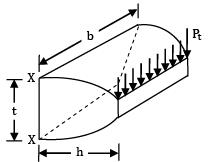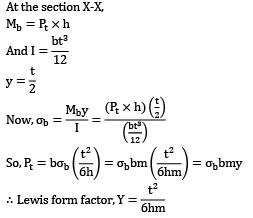Courses

# Test:Design Of Gears (Level - 1)

## 10 Questions MCQ Test Engineering Mechanics | Test:Design Of Gears (Level - 1)

Description
This mock test of Test:Design Of Gears (Level - 1) for Mechanical Engineering helps you for every Mechanical Engineering entrance exam. This contains 10 Multiple Choice Questions for Mechanical Engineering Test:Design Of Gears (Level - 1) (mcq) to study with solutions a complete question bank. The solved questions answers in this Test:Design Of Gears (Level - 1) quiz give you a good mix of easy questions and tough questions. Mechanical Engineering students definitely take this Test:Design Of Gears (Level - 1) exercise for a better result in the exam. You can find other Test:Design Of Gears (Level - 1) extra questions, long questions & short questions for Mechanical Engineering on EduRev as well by searching above.
QUESTION: 1

Solution:
QUESTION: 2

Solution:
QUESTION: 3

### Lewis equation in spur gear design applied to

Solution:

Lewis equation in spur gear design is applied weaker of the pinion or gear.

QUESTION: 4

In an involute gear, the base circle must be __________

Solution:
QUESTION: 5

What is the profile addendum of a cycloidal gear tooth?

Solution:
QUESTION: 6

Solution:

Gear teeth is made harder to avoid wear and also for improved strength.

QUESTION: 7

The minimum required center distance of two mating spur gears is decided based on

Solution:

The minimum required center distance of two mating spur gears is decided based on bending strength of the gear material.

QUESTION: 8

A gear tooth is shown in the figureIf m is the module, then the Lewis form factor is (assuming tooth as cantilever)

Solution:

At the section X-X,

Mb = Pt x hQUESTION: 9

In which of the following, the service factor is not 1.

Solution:

T - θ diagram is constant for all, except IC engine case. This variation of torque gives rise to

CS ≠ 1

QUESTION: 10

Which one of the following properties is required for an involute spur gear tooth to satisfy law of gearing

Solution:

Hardness is required to prevent wear and maintain same involute tooth profile throughout the time.